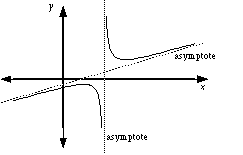index: click on a letter A B C D E F G H I J K L M N O P Q R S T U V W X Y Z A to Z index index: subject areas numbers & symbols sets, logic, proofs geometry algebra trigonometry advanced algebra & pre-calculus calculus advanced topics probability & statistics real world applications multimedia entrieswww.mathwords.com about mathwords website feedback

 Asymptote A line or curve that the graph of a relation approaches more and more closely the further the graph is followed. Note: Sometimes a graph will cross a horizontal asymptote or an oblique asymptote. The graph of a function, however, will never cross a vertical asymptote.